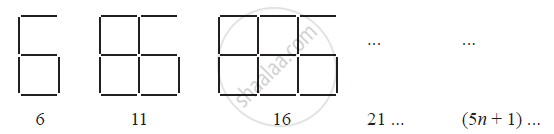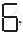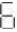# Observe the Patterns of Digits Made from Line Segments of Equal Length. You Will Find Such Segmented Digits on the Display of Electronic Watches Or Calculators. - Mathematics

Observe the patterns of digits made from line segments of equal length. You will find such segmented digits on the display of electronic watches or calculators.If the number of digits formed is taken to be n, the number of segments required to
form n digits is given by the algebraic expression appearing on the right of each pattern.

How many segments are required to form 5, 10, 100 digits of the kind −#### Solution

It is given that the number of segments required to form n digits of the kindis (5n + 1)

Number of segments required to form 5 digits = (5 × 5 + 1)

= 25 + 1 = 26

Number of segments required to form 10 digits = (5 × 10 + 1)

= 50 + 1 = 51

Number of segments required to form 100 digits = (5 × 100 + 1)

= 500 + 1 = 501

Concept: Use of Variables in Common Rules
Is there an error in this question or solution?
Chapter 12: Algebraic Expressions - Exercise 12.4 [Page 246]

#### APPEARS IN

NCERT Class 7 Maths
Chapter 12 Algebraic Expressions
Exercise 12.4 | Q 1.1 | Page 246
Share